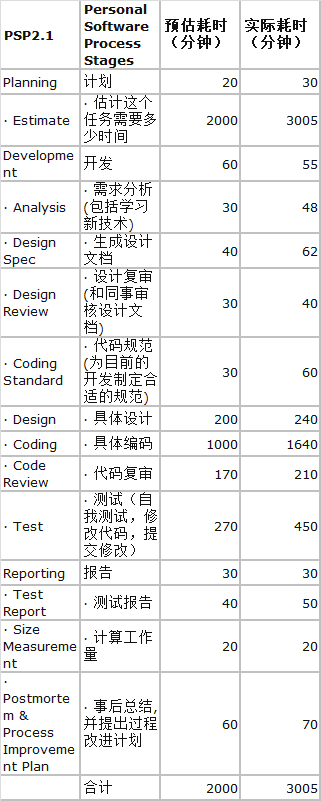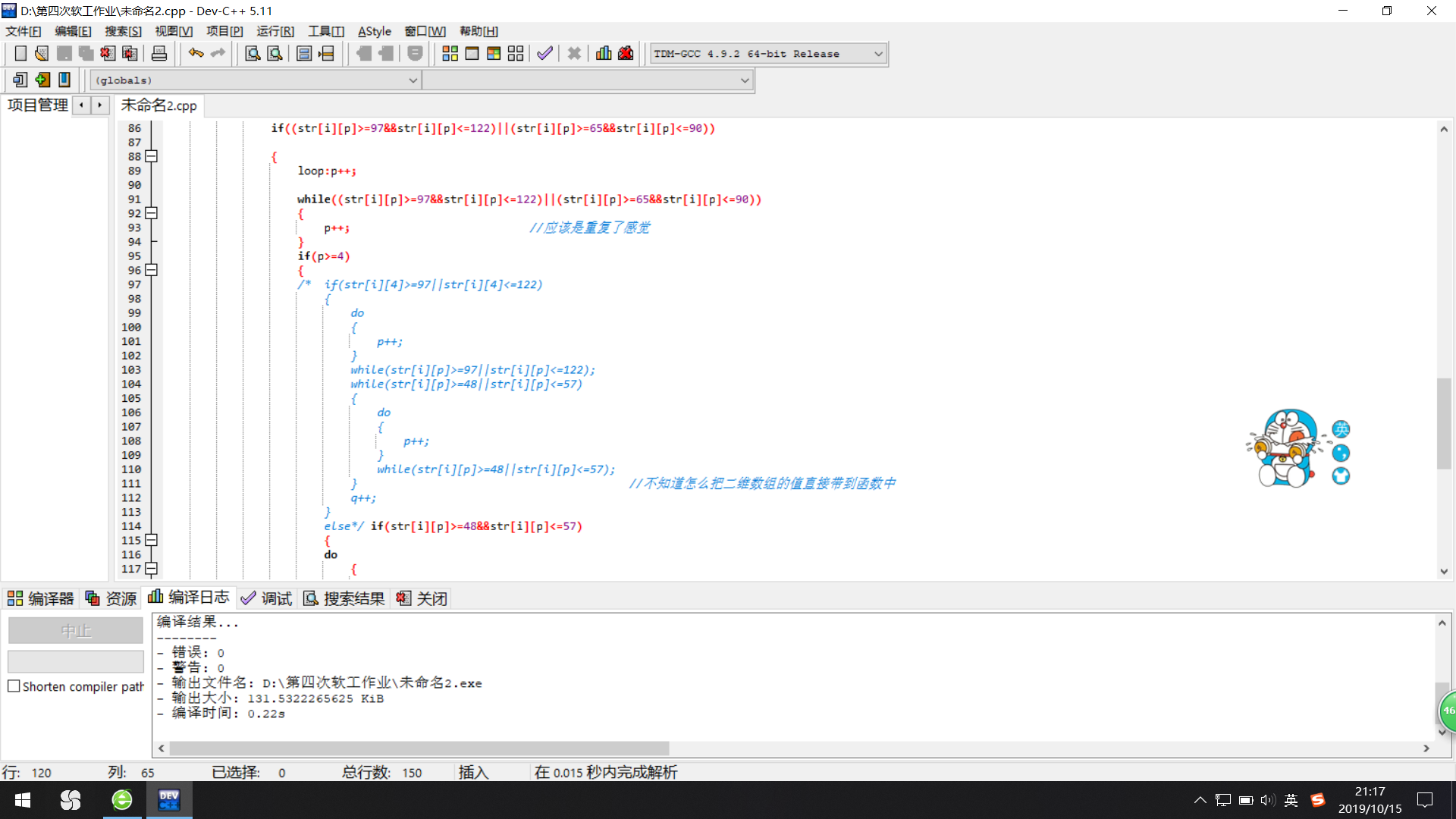git地址 https://github.com/npcccc1/WordCount 伙伴 徐睿201831061409 伙伴博客 https://www.cnblogs.com/codingbyjusticexu/

# 一、PSP表格# 二、代码实现

和伙伴选择了不同的语言来完成这项工作（c与c++），所以用了两个仓库放不同的项目。由于并不在一个寝室，具体的代码是每人自己敲的。事后的测试、代码规范与调整则在商讨之后进一步完善。

int main()                                                      //简单的主函数先放上
{
FILE *fpa;
FILE *fpb;
if ((fpa = fopen("D:\\wordCount.txt", "r")) == NULL)
{
printf("Failure to open wordCount.txt!\n");
exit(0);
}
if ((fpb = fopen("D:\\Countresult.txt", "w")) == NULL)
{
printf("Failure to open wordCount.txt!\n");
exit(0);
}
countchar(fpa,fpb);
wordcount(fpa,fpb);
fclose(fpa);
fclose(fpb);
return 0;
}


　　　　　void countchar(FILE *a,FILE *b)                    //统计字符函数
{                                                  //ps:一开始想成了输出a到z 26个字母的个数...
char c;
int  d=0;
int  i=0;
int  p=0;
int  q=0;
while(!feof(a))
{
c=fgetc(a);
if(c>=48&&c<=57)
{
d++;
}
else if(c>=65&&c<=90)
{
i++;
}
else if(c>=97&&c<=122)
{
p++;
}
else
{
q++;
}
}
rewind(a);
fprintf(b,"0--9\t%d\nA--Z\t%d\na--z\t%d\nSeparator\t%d\n",d,i,p,q);
}


	void wordcount(FILE *a,FILE *b)
{
int  i;
int  q=0;
char str[N][N];
while(!feof(a))
{
for(i=0;;i++)
{
int p=0;
fgets(str[i],N,a);

if((str[i][p]>=97&&str[i][p]<=122)||(str[i][p]>=65&&str[i][p]<=90))

{
loop:p++;                        //按理说不应该加goto实在不想再用循环了

while((str[i][p]>=97&&str[i][p]<=122)||(str[i][p]>=65&&str[i][p]<=90))
{
p++;
}
if(p>=4)
{
if(str[i][p]>=48&&str[i][p]<=57)
{
do
{
p++;
}
while(str[i][p]>=48&&str[i][p]<=57);
q++;
goto loop;
}
q++;
}

}

fprintf(b,"%d\t",q);
}
}

}


# 三、代码规范

经讨论后，规范详见此链接。对照后看了自己的代码发现一个小坏习惯之前没注意的——写函数时总是空出一个制表符，导致在复杂的循环中“{}”往往因为开头的这个Tab而向后缩进，显得前面一大片空白，影响美观。

还有便是变量的取名不大规范，往往是自己随心所欲地取。

# 四、代码复审

与其说是复审，其实更像在写中改。开始编译没什么问题还是很开心的，但打开文件发现没有打印出任何东西。上一个四则运算也是遇到了这种情况，上次是因为&&与||有一次打错，导致循环卡到了一处没输出任何算式。这是一开始第一次传到github中的代码，循环太多要处理带数字或其他符号的字符串是不是单词，让脑子有点乱，一开始没输出也是这个的原因。此外也有零零散散的小错误，也是要多看几遍自己就能检查出来。为了效率，也是因为用的不同的语言不同的方法，没再让同伴互查。

# 五、性能分析

不知道为什么复制过去vs里面说fopen可能不安全，编译不出来测试不出来。

# 六、总结与反思

对c语言的面向过程深有了感触，再加上自己没有细分模块与函数导致出错多、效率低下，感觉还是c++封装及模块的划分比c语言简单。但是结对确实是提供了思路即使我们交流代码不大详细，往往在qq上交流一些问题和进度及自己的思路，可以明显感到1+1>2，